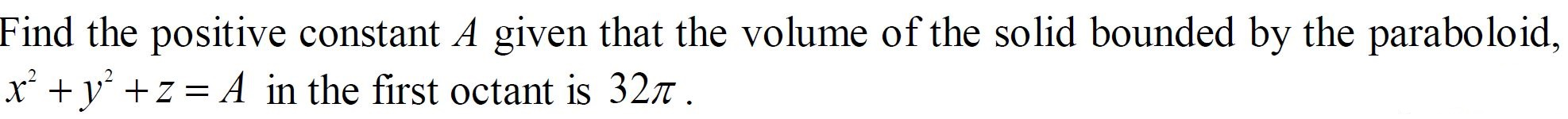# Find the positive constant A given that the volume of the solid bounded by the paraboloid, x +y +z = A in the first octant is 327 .

Question

Find the positive constant A given that the volume of the solid bounded by the paraboloid, x2 + y2 +z = A  in the first octant is 32π .help_outlineImage TranscriptioncloseFind the positive constant A given that the volume of the solid bounded by the paraboloid, x +y +z = A in the first octant is 327 . fullscreen

### Want to see this answer and more?

Experts are waiting 24/7 to provide step-by-step solutions in as fast as 30 minutes!*

*Response times may vary by subject and question complexity. Median response time is 34 minutes for paid subscribers and may be longer for promotional offers.
Tagged in
Math
Calculus

### Integration